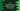# C++ program to find the second highest number in an array# Introduction :

In this tutorial, we will learn how to find the second-highest number in an array in C++. The program will take the array elements as input from the user. It will then find out the second-highest element and print it out.

We are using one for loop to find out the number. Following algorithm we will use in this program :

## Algorithm :

We need to find the second highest number in an array. The program will scan through each element of the array one by one. It will keep two variables to hold the highest and the second highest number.

For each element, it will check if it is bigger than the highest number or not. If yes, it will update both highest and the second highest numbers. At the end of the loop, we can print the second highest number of the array.

## C++ program :

``````#include <iostream>
using namespace std;

int main()
{
//1
int total;
int highest;
int secondHighest;

//2
cout << "Enter total number of elements : "; cin >> total;

//3
int arr;

//4
for (int i = 0; i < total; i++)
{
cout << "Enter array element : " << endl; cin >> arr[i];
}

//5
highest = arr;
secondHighest = arr;

//6
for (int i = 1; i < total; i++) { //7 if (arr[i] > highest)
{
secondHighest = highest;
highest = arr[i];
}
}

//8
cout << "Second highest number in the given array is : " << secondHighest << endl;
}``````

### Explanation :

The commented numbers in the above program denote the step numbers below :

1. Create three integer variables to hold the total number of elements, highest value and second-highest values.
2. Ask the user to enter the total number of elements. Read and store it in the variable total.
3. Create one integer array to hold the user input values.
4. Run one for loop. Ask the user to enter the array elements one by one. Read and enter each value in the array arr.
5. Assign the first element of the array to the variables highest and secondHighest.
6. Run one for loop to iterate through the array elements one by one.
7. Check for each element. If the element is greater than the value stored in highest, assign the value of highest to secondHighest and assign this value to highest. i.e. we are updating the value of the second highest element while iterating.
8. Finally, print out the second-highest element in the array.

### Sample Example :

``````Enter total number of elements : 5
Enter array element :
3
Enter array element :
55
Enter array element :
43
Enter array element :
56
Enter array element :
122
Second highest number in the given array is : 56``````